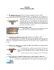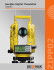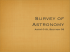TACHEOMETRY

Transcription

TACHEOMETRY
TACHEOMETRY
Unit 1
Tacheometry
• Defined as a procedure of obtaining horizontal distances and differences in elevation based on the optical geometry of the instrument employed
of the instrument employed
• compared to taping and differential leveling, the g,
distances and elevations obtained by tacheometric methods are usually of a lower
methods are usually of a lower degree of accuracy
Uses of Tacheometric Methods
Uses of Tacheometric Methods
• Check the more accurate taped distances to uncover p
errors or mistakes
• Determine differences of elevation between points
• Carry lines of levels where low order accuracy is required
• Measure the lengths of traverse lines
M
th l th f t
li
• Compile planimetric and topographic maps
• Complete field survey for photographic map
Complete field survey for photographic map
• Locate details for hydrographic survey
• this method employs p y
the sighting telescope of an instrument in reading a small angle
reading a small angle along a vertical plane and in determining the g
length which the angle subtends on a graduated rod held
graduated rod held vertical on the distant point
600 Greek units, or 184
m 93 cm (606 ft 9 in) by
present-day international
standards
 the term is now applied to the cross hairs and
rod used in making measurements, as well as to
the method itself
 the equipment for stadia measurements
consists of a telescope with two horizontal hairs
p y byy the
 distances can be measured veryy rapidly
Since ab is equal to a’b’, by similar triangles
q
y
g
f/i = d/s
And
d = (f/i)s
also
D = d + (f + c)
D = (f/i)s + C
D = Ks + C
 the stadia constant, the distance from the center of
principal
p focus
the instrument to the p
 the quantity is composed of the the focal length (f),
which remains constant, and the distance (c) from the
center of the instrument to the center of the objective
lens
 in the older instruments,
instruments C varies from about 0.18
0 18 to
0.43 m
 in present
present‐day
day surveying instruments, C may be
considered 0.30 for external‐focusing telescopes, 0 for
internal‐focusing telescopes
 the ratio f/I is called the stadia interval factor
 for any given instrument, the value remains
constant and depends only on the spacing
 the most common value of K is 100
Sample Problem
Sample Problem
A theodolite is set up at one end of a level base line 150 0m long The line is marked by stakes at
line 150.0m long. The line is marked by stakes at every 30.0m and a stadia rod is held at each stake. The stadia intercept at each location is observed as follows: 0.302, 0.600, 0.899, 1.207, and 1.506 meters, respectively. Compute the stadia interval f
factor (K) for each distance and also determine (K) f
h di
d l d
i
the average value of K.
Solution
k=D/s
k1 = 30/ 0.302 = 99.3
k2 = 60/ 0.600 = 100.0
60/ 0 600 00 0
k3 = 90/ 0.899 = 100.1
k4 = 120/1.207 = 99.4
k5 150/1 506 = 99.6
99 6
5 = 150/1.506 kave = 99.7 Sample Problem
Sample Problem
An automatic level with an internal focusing telescope was set up somewhere at mid‐length
telescope was set up somewhere at mid
length of of
a long‐span steel bridge. The rod readings tabulated below were observed on a stadia rod held successively at the vicinity of the concrete abutments in the southern and northern approaches of the bridge. If the stadia interval h
f h b id If h
di i
l
factor of the instrument is 98.5, determine the length of the bridge
length of the bridge.
Sample Problem
Sample Problem
Rod
Position
Rod at
Southern
Approach
Rod at
Northern
Approach
Upper (a)
2.98 m
Middle (c)
1.68 m
Lower (b)
0.38 m
3 54 m
3.54
2 02 m
2.02
0 49 m
0.49
Solution
ss= 2.98 = 2 98 ‐ 0.38 =2.60
0 38 =2 60
Ds= 98.5 * 2.60 = 256.1 m
sn= 3.54 ‐
3
0 9 30
0.49 = 3.05
Dn= 98.5 * 3.05 = 300.4 m
D = 256.1 + 300.4 = 556.5 m
ID = ks cos α + C (eq 2)
ID = ks cos α
+ C (eq 2)
2 α + C cos α
2 HD = ks cos
k
C
( 3)
(eq 3)
VD = ks cos α sin α + C sin α (eq 4)
DE = HI + VD ‐ RR
Sample Problem
Sample Problem
The following data were obtained by stadia observations: vertical angle = +9º25’, upper stadia hair and lower stadia hair readings are 2.352m and 0.995m, respectively. The stadia interval factor is known to be 99.0 and C is 0.381m. The height of the instrument above the instrument station (point A) is 1.496m and rod reading is taken at 1.589m. Determine the g
following:
a) horizontal, vertical, and inclined distances by exact stadia formulas
b) elevation of the point sighted (point B) is the elevation of
b) elevation of the point sighted (point B) is the elevation of point A is 776.545m.
c) difference in elevation between the two points.

Literature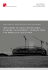PDF Lot View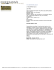playing-the-field-music-week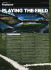Engineering 9 – Statics Sample Test for Exam #2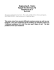The Shape of the Earth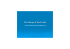Pectacular TW Lewis Home With Lots Of Upgrades!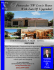LEVELS Final as of March 19, 2006